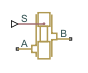# Annular Orifice

(To be removed) Hydraulic variable orifice created by circular tube and round insert

The Hydraulics (Isothermal) library will be removed in a future release. Use the Isothermal Liquid library instead. (since R2020a)

•Libraries:
Simscape / Fluids / Hydraulics (Isothermal) / Orifices

## Description

The Annular Orifice block annular leakage in a fully-developed laminar flow created by a circular tube and a round insert in an isothermal liquid network. The insert can be located off-center from the tube by an eccentricity value.The flow rate is computed using the Hagen-Poiseuille equation (see ):

`$q=\frac{\pi R{\left(R-r\right)}^{3}}{6\nu \rho L}·\left(1+\frac{3}{2}{\epsilon }^{2}\right)·p$`

`$\epsilon =\frac{e}{R-r}$`

where

 `q` Flow rate `p` Pressure differential `R` Orifice radius `r` Insert radius `L` Overlap length ε Eccentricity ratio `e` Eccentricity ρ Fluid density ν Fluid kinematic viscosity

Use this block to simulate leakage path in plungers, valves, and cylinders.

The block positive direction is from port A to port B. This means that the flow rate is positive if it flows from A to B and the pressure differential is determined as $\Delta p={p}_{\text{A}}-{p}_{\text{B}},$. Positive signal at the physical signal port `S` increases or decreases the overlap, depending on the value of the parameter Orifice orientation.

## Assumptions and Limitations

Fluid inertia is not taken into account.

## Ports

### Input

expand all

Physical signal port that controls the insert displacement.

### Conserving

expand all

Hydraulic conserving port associated with the orifice inlet.

Hydraulic conserving port associated with the orifice outlet.

expand all